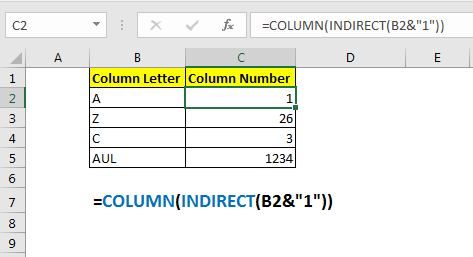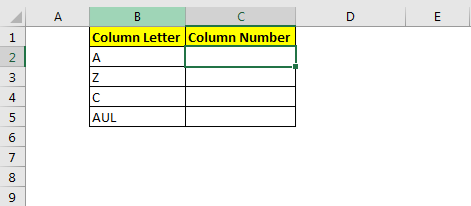# How To Convert Column Letter to Number in Excel

Earlier, we learnt how to convert the column number to the letter. But how do we convert column letter to number in excel? In this article, we learn to convert excel column to number.So we have a function named COLUMN that returns the column number of supplied reference. We will use the COLUMN function with INDIRECT function to get the column number from a given column letter.
Generic Formula to convert letters to numbers in excel

=COLUMN(INDIRECT(col_letter & “1”))

Col_letter: it is the reference of the column letter of which you want to get column number.
Var2:

Let’s see an example to make things clear.
Example: Create an excel column letter to number converter
Here we have some column letters in B2:B5. We want to get corresponding column number (1, 2, 3, etc.) from that given letter (A, B, C, etc.).Apply the above generic formula here to get column number of a given letter.

=COLUMN(INDIRECT(B2&"1"))

Copy it down. You will have the column number of the given column letter in B2:B5.How does it work?
Well, it is very simple. The idea is to get the first cell's reference from the given column number. And then use COLUMN function to get the column number of a given column letter.
Here, INDIRECT(B2&"1") translates to INDIRECT(“A1"). This then gives us the cell reference of A1.
Eventually, we get COLUMN(A1), which then returns the column number of a given column letter.

So yeah, this is how to convert a column letter into a column index number. This is quite easy. Let me know if you have any doubt regarding this function or any other function in advanced excel. The comments section is all yours.

Related Articles:

How to Convert Excel Column Number To Letter

Popular Articles:

The VLOOKUP Function in Excel

COUNTIF in Excel 2016

How to Use SUMIF Function in Excel

Terms and Conditions of use

The applications/code on this site are distributed as is and without warranties or liability. In no event shall the owner of the copyrights, or the authors of the applications/code be liable for any loss of profit, any problems or any damage resulting from the use or evaluation of the applications/code.